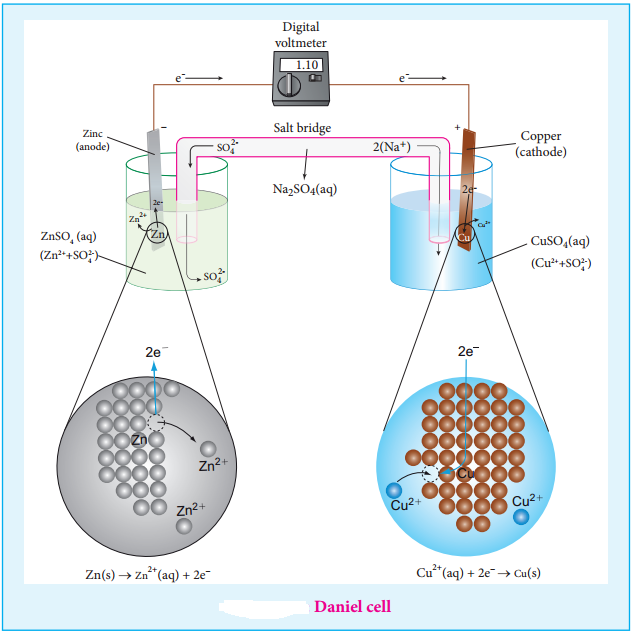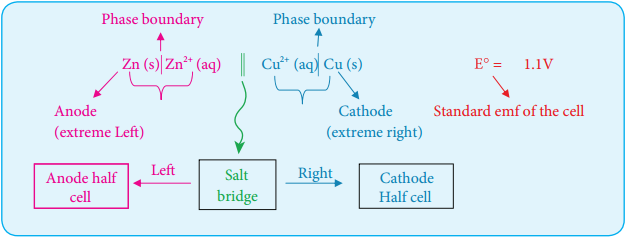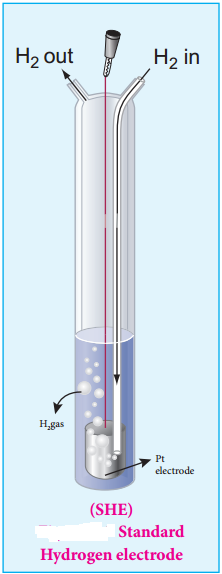Find free online Chemistry Topics covering a broad range of concepts from research institutes around the world.

## Electrochemical Cell

Electrochemical cell is a device which converts chemical energy into electrical energy and vice versa. It consists of two separate electrodes which are in contact with an electrolyte solution. Electrochemical cells are mainly classified into the following two types.

1. Galvanic Cell (Voltaic Cell):

It is a device in which a spontaneous chemical reaction generates an electric current i.e., it converts chemical energy into electrical energy. It is commonly known as a battery.

2. Electrolytic Cell:

It is a device in which an electric current from an external source drives a nonspontaneous reaction i.e., it converts electrical energy into chemical energy.

Galvanic Cell

We have already learnt in XI standard that when a zinc metal strip is placed in a copper sulphate solution, the blue colour of the solution fades and the copper is deposited on the zinc strip as red – brown crust due to the following spontaneous chemical reaction.

Zn(s) + CuSO4(aq) → ZnSO4(aq) + Cu(s)

The energy produced in the above reaction is lost to the surroundings as heat.

In the above redox reaction, Zinc is oxidised to Zn2+ ions and the Cu2+ ions are reduced to metallic copper.
The half reactions are represented as below.

Zn(s) → Zn2+(aq) + 2e (oxidation)
Cu2+(aq) + 2e → Cu(s) (reduction)

If we perform the above two half reactions separately in an apparatus as shown in figure 9.5, some of the energy produced in the reaction will be converted into electrical energy. Let us understand the function of a galvanic cell by considering Daniel cell as an example. It uses the above reaction for generation of electrical energy. The separation of half reaction is the basis for the construction of Daniel cell. It consists of two half cells.

Oxidation Half Cell

A metallic zinc strip that dips into an aqueous solution of zinc sulphate taken in a beaker, as shown in Figure 9.5.Reduction Half Cell

A copper strip that dips into an aqueous solution of copper sulphate taken in a beaker, as shown in Figure 9.5.Joining the Half Cells

The zinc and copper strips are externally connected using a wire through a switch (k) and a load (example: volt meter). The electrolytic solution present in the cathodic and anodic compartment are connected using an inverted U tube containing a agar-agar gel mixed with an inert electrolytes such as KCl, Na2SO4 etc., The ions of inert electrolyte do not react with other ions present in the half cells and they are not either oxidised (or) reduced at the electrodes. The solution in the salt bridge cannot get poured out, but through which the ions can move into (or) out of the half cells.

When the switch (k) closes the circuit, the electrons flows from zinc strip to copper strip. Ths is due to the following redox reactions which are taking place at the respective electrodes.

Anodic Oxidation

The electrode at which the oxidation occurs is called the anode. In Daniel cell, the oxidation take place at zinc electrode, i.e., zinc is oxidised to Zn2+ ions by loosing its electrons. The Zn2+ ions enter the solution and the electrons enter the zinc metal, then flow through the external wire and then enter the copper strip. Electrons are liberated at zinc electrode and hence it is negative (- ve).

Zn(s) → Zn2+(aq) + 2e (loss of electron-oxidation)

Cathodic Reduction

As discussed earlier, the electrons flow through the circuit from zinc to copper, where the Cu2+ ions in the solution accept the electrons, get reduced to copper and the same get deposited on the electrode. Here, the electrons are consumed and hence it is positive (+ ve).

Cu2+ + 2e → Cu (s) (gain of electron-reduction)

Salt Bridge

The electrolytes present in two half cells are connected using a salt bridge. We have learnt that the anodic oxidation of zinc electrodes results in the increase in concentration of Zn2+ in solution. i.e., the solution contains more number of Zn2+ ions as compared to SO42- and hence the solution in the anodic compartment would become positively charged.

Similarly, the solution in the cathodic compartment would become negatively charged as the Cu2+ ions are reduced to copper i.e., the cathodic solution contain more number of SO42- ions compared to Cu2+.

To maintain the electrical neutrality in both the compartments, the non reactive anions Cl (from KCl taken in the salt bridge) move from the salt bridge and enter into the anodic compartment, at the same time some of the K+ ions move from the salt bridge into the cathodic compartment.

Completion of Circuit

Electrons flow from the negatively charged zinc anode into the positively charged copper cathode through the external wire, at the same time, anions move towards anode and cations are move towards the cathode compartment. This completes the circuit.

Consumption of Electrodes

As the Daniel cell operates, the mass of zinc electrode gradually decreases while the mass of the copper electrode increases and hence the cell will function until the entire metallic zinc electrode is converted in to Zn2+ or the entire Cu2+ ions are converted in to metallic copper.

Unlike Daniel cell, in certain cases, the reactants (or) products cannot serve as electrodes and in such cases insert electrode such as graphite (or) platinum is used which conducts current in the external circuit.

Galvanic Cell Notation

The galvanic cell is represented by a cell diagram, for example, Daniel cell is represented as

Zn (s)|Zn2+(aq)||Cu2+(aq)|Cu (s)

In the above notation, a single vertical bar (|) represents a phase boundary and the double vertical bar (||) represents the salt bridge. The anode half cell is written on the left side of the salt bridge and the cathode half cell on the right side.

The anode and cathode are written on the extreme left and extreme right, respectively. The emf of the cell is written on the right side after cell diagram.Example

The net redox reaction of a galvanic cell is given below
2 Cr (s) + 3Cu2+(aq) → 2Cr3+ (aq) + 3Cu (s)

Write the half reactions and describe the cell using cell notation.
Anodic oxidation 2Cr (s) → 2Cr3+ (aq) + 6e ….. (1)
Cathodic reduction : 3Cu2+ (aq) + 6e → 3 Cu (s) ….. (2)

Cell Notation is
Cr (s)|Cr 3+(aq)||Cu2+(aq)|Cu(s)

Emf of a Cell

We have learnt that when two half cells of a Daniel cell are connected, a spontaneous redox reaction will take place which results in the flow of electrons from anode to cathode. The force that pushes the electrons away from the anode and pulls them towards cathode is called the electromotive force (emf) (or) the cell potential. The SI unit of cell potential is the volt (v).

When there is one volt difference in electrical potential between the anode and cathode, one joule of energy is released for each columb of charge that moves between them.

i.e., 1J = 1C × 1V ….. (9.18)

The cell voltage depends on the nature of the electrodes, the concentration of the electrolytes and the temperature at which the cell is operated. For example

At, 25°C , The emf of the below mentioned Daniel cell is 1.107 Volts

Zn (s)|Zn2+(aq, 1M)||Cu2+(aq, 1M)|Cu (s) E° = 1.107 V0lts

Measurement of Electrode Potential

The overall redox reaction can be considered as the sum of two half reactions i.e., oxidation and reduction. Similarly, the emf of a cell can be considered as the sum of the electrode potentials at the cathode and anode,

Ecell = (Eox)anode + (Ered)cathode ….. (9.19)

Here, (Eox)anode represents the oxidation potential at anode and (Ered)cathode represents the reduction potential at cathode. It is impossible to measure the emf of a single electrode, but we can measure the potential difference between the two electrodes (Ecell) using a voltmeter.

If we know the emf of any one of the electrodes which constitute the cell, we can calculate the emf of the other electrode from the measured emf of the cell using the expression (9.19). Hence, we need a reference
electrode whose emf is known.

For that purpose, Standard Hydrogen Electrode (SHE) is used as the reference electrode. It has been assigned an arbitrary emf of exactly zero volt. It consists of a platinum electrode in contact with 1M HCl solution and 1 atm hydrogen gas. The hydrogen gas is bubbled through the solution at 25°C as shown in the figure 9.6. SHE can act as a cathode as well as an anode.

The Half cell reactions are given below.

If SHE is used as a cathode, the reduction reaction is
2H+(aq, 1M) + 2e → H2(g, 1 atm) E° = 0 volt

If SHE is used as an anode, the oxidation reaction is
H2(g, 1 atm) → 2H+(aq, 1M) + 2e E° = 0 voltIllustration

Let us calculate the reduction potential of zinc electrode dipped in zinc sulphate solution using SHE.

Step: 1

The following galvanic cell is constructed using SHE
Zn (s) | Zn2+(aq, 1M)|| H+(aq, 1M)| H2 (g, 1atm)| Pt (s)

Step: 2

The emf of the above galvanic cell is measured using a volt meter. In this case, the measured emf of the above galvanic cell is 0.76V.

Calculation
We know that,

cell = (E°ox)zn|zn2++ (E°red)SHE [From equation (9.19)]
cell = 0.76 and (E°red)SHE = 0V . Substitute these values in the above equation⇒ 0.76V = (E°ox)zn|zn2+ + 0V
⇒ (E°ox)zn|zn2+ = 0.76V

This oxidation potential corresponds to the below mentioned half cell reaction which takes place at the cathode.

Zn → Zn2++ 2e (Oxidation)

The emf for the reverse reaction will give the reduction potential
Zn2++2e → Z ; E° = – 0.76V
∴ (Eo°red)zn2+|zn = – 0.76V.